## Worksheet 03.4: Descriptive Measures Again

The task here is to come up with descriptive measures for the data in Table 1.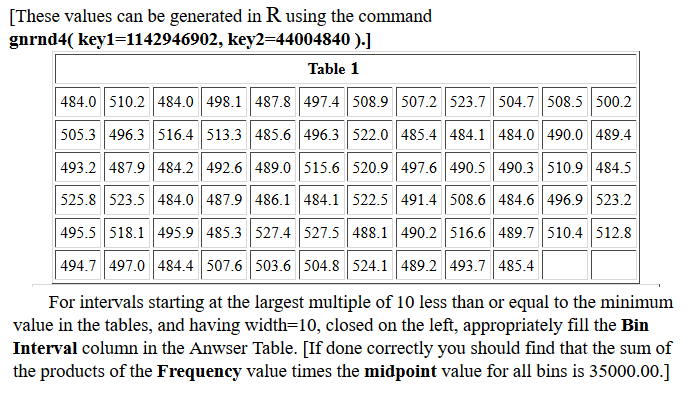This page assumes that you have read through earlier pages and that you have mastered the steps that we use to set up our work. To that end we will assume that we have
1. inserted our USB drive,
2. created a directory called `worksheet034` on that drive
3. have copied `model.R` from our root folder into our new folder,
4. have renamed that new copy of the file to the name `ws34.R`, and
5. have double clicked on that file to open RStudio.
The result should be a window pretty much identical to the one shown in Figure 1. {Recall that the images shown here may have been reduced to make a printed version of this page a bit shorter than it woud otherwise appear. In most cases your browser should allow you to right click on an image and then select the option to View Image in order to see the image in its original form.}

Figure 1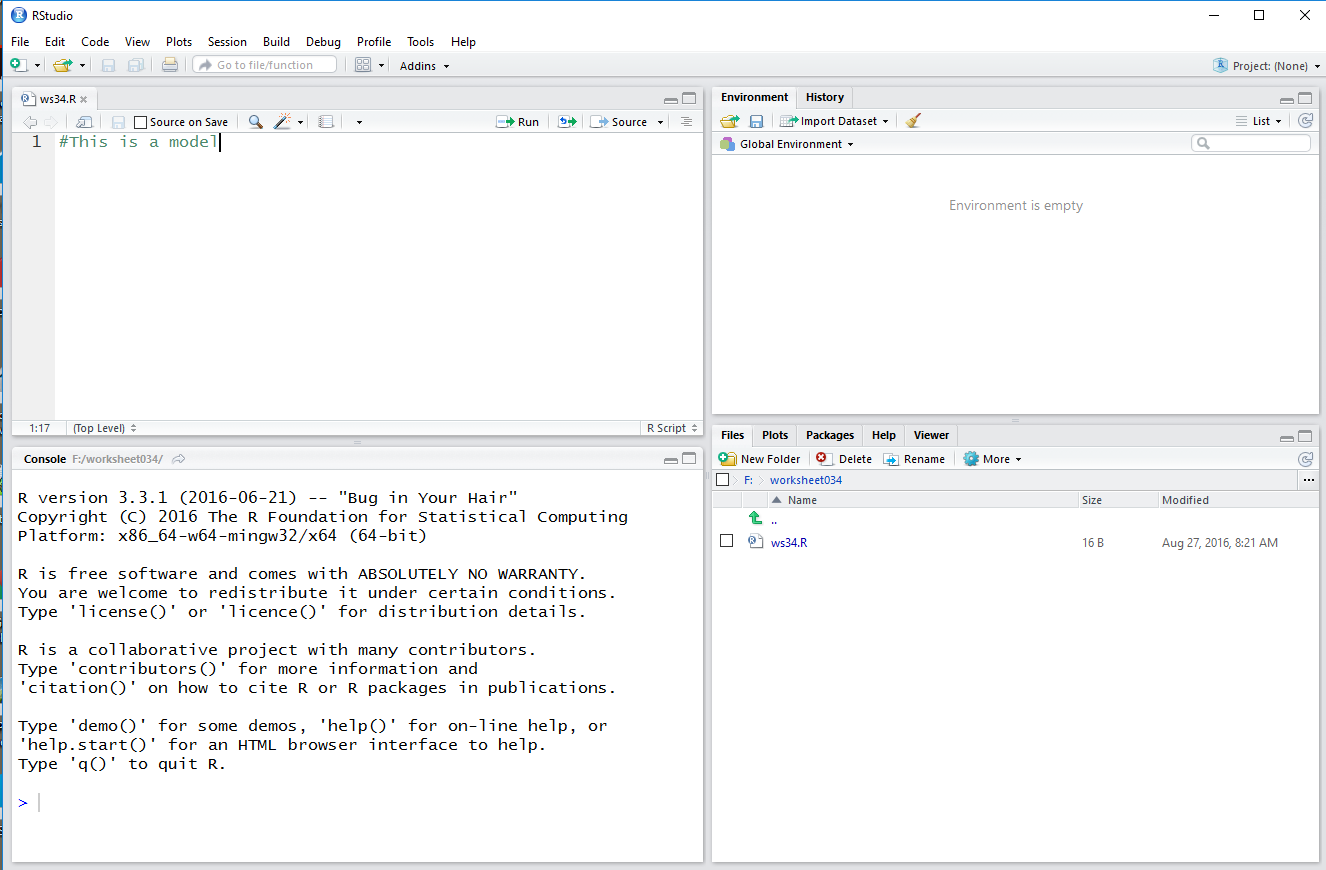Much of the discussion that would be given here has been included in the comments in our `ws34.R` file. Therefore, it is important to read those comments. We start by loading the various functions we will need. Then we generate the data values and examine them to be sure that we have the correct values.

Figure 2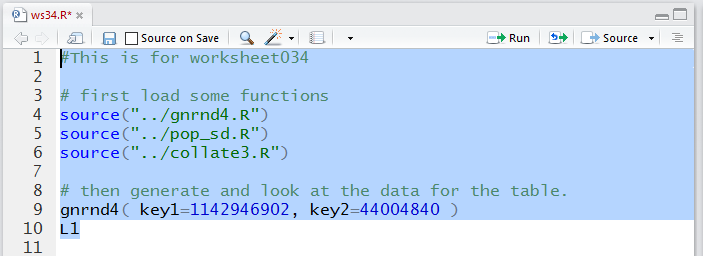The console view of those commands:

Figure 3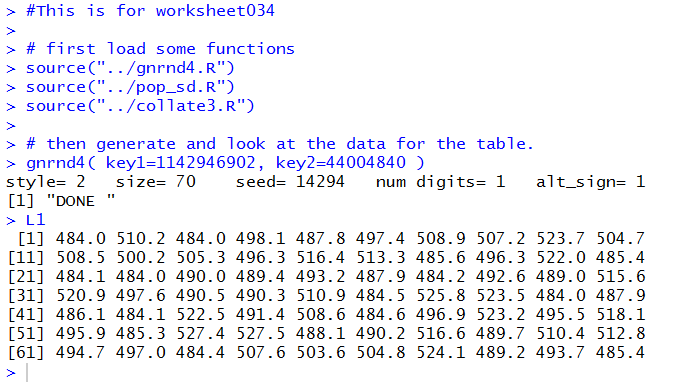The we add the commands to generate many of our descriptive statistics.

Figure 4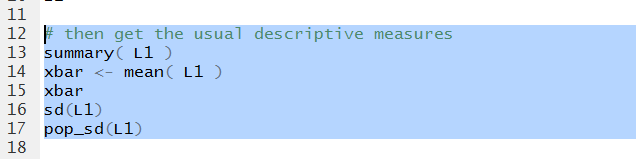In Figure 5 we can see all of those values.

Figure 5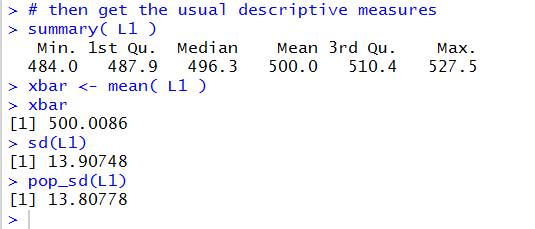Now we want to generate the frequency table. We are given specific direction on the start of the first bin and the width of the bins. Note that we are storing the generated table in the variable `ft`, then displaying that table in the Console pane, then displaying it in a better format via the `View(ft)` command.

Figure 6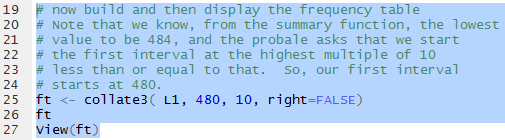In Figure 7 we can read the frequency table values in the Console pane.

Figure 7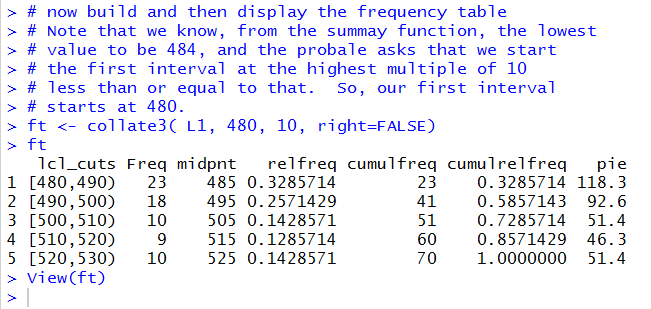Figure 8 shows the same values, but in a more fancy display.

Figure 8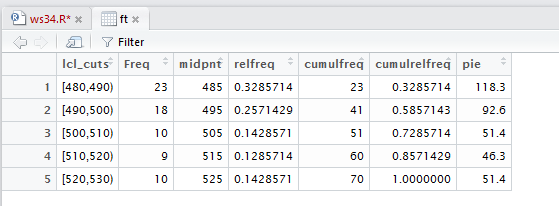As stated in the comment shown in Figure 9, we really could just re-enter the five values for the frequencies and the five vales for the midpoints. However, since they are already in the system, after all we did see them in the table, we can go look for them. The command `str(ft)` has R display the structure of the data frame called `ft`.

Figure 9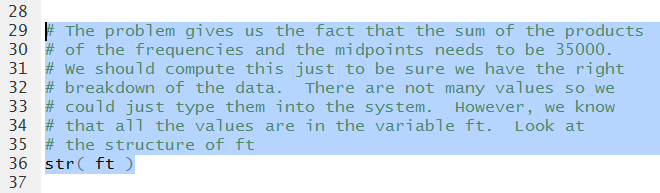In Figure 10 we note that the frequency values are stored in `ft\$Freq` while the midpoint values are stored in `ft\$midpnt`.

Figure 10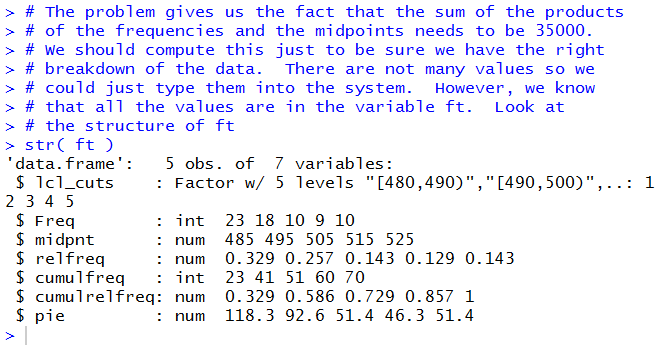We use those two names just to prove to ourselves that they hold the values that we need.

Figure 11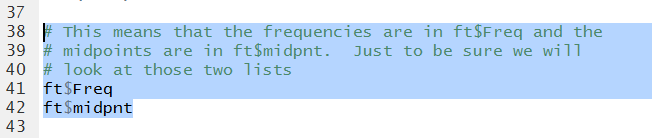A run of those commands gives the output shown in Figure 12.

Figure 12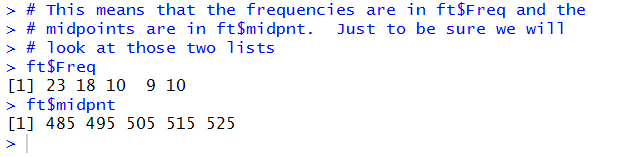That is good enough for us so we can use the two variables to generate our desired sum.

Figure 13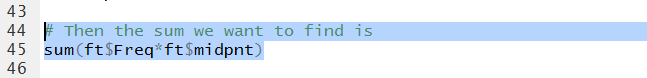Figure 14 shows the resulting computed value. It checks with the value given in the problem so we feel confident that we have done the right thing.

Figure 14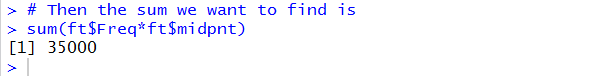In Figure 15 we form the command to generate a bax and whisker plot.

Figure 15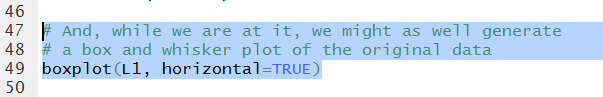Running that command does not provide much in the Console pane, shown in Figure 16.

Figure 16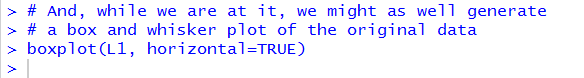However, in the Plot pane we find quite an acceptable chart.

Figure 17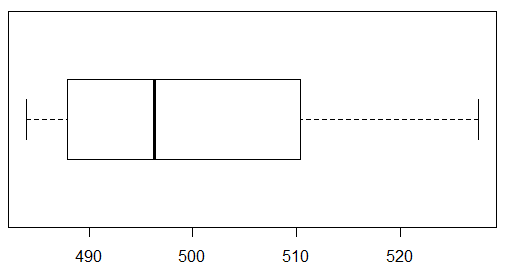The `hist(L1)` command will produce a default histogram.

Figure 18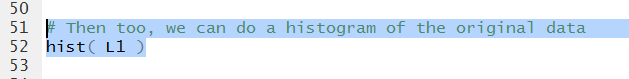That histogram appears in the Plot pane as shown in Figure 19.

Figure 19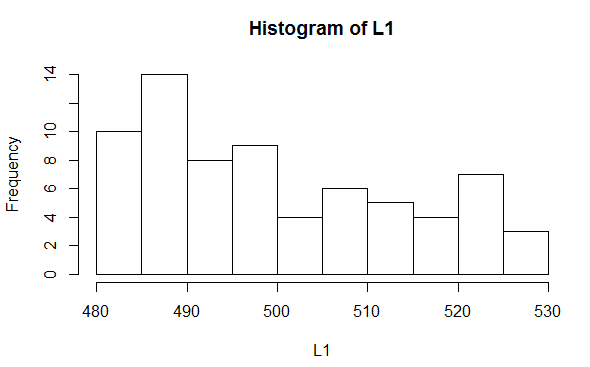All that is left to do is to save our `ws24.R` file and then type the `q()` command in the Console, respond to the prompt with `y`, and press the Enter key to close our RStudio session.

Figure 20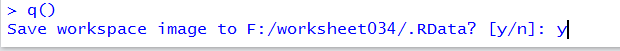Here is a listing of the complete contents of the `ws34.R` file:
```#This is for worksheet034

source("../gnrnd4.R")
source("../pop_sd.R")
source("../collate3.R")

# then generate and look at the data for the table.
gnrnd4( key1=1142946902, key2=44004840 )
L1

# then get the usual descriptive measures
summary( L1 )
xbar <- mean( L1 )
xbar
sd(L1)
pop_sd(L1)

# now build and then display the frequency table
# Note that we know, from the summary function, the lowest
# value to be 484, and the problem asks that we start
# the first interval at the highest multiple of 10
# less than or equal to that.  So, our first interval
# starts at 480.
ft <- collate3( L1, 480, 10, right=FALSE)
ft
View(ft)

# The problem gives us the fact that the sum of the products
# of the frequencies and the midpoints needs to be 35000.
# We should compute this just to be sure we have the right
# breakdown of the data.  There are not many values so we
# could just type them into the system.  However, we know
# that all the values are in the variable ft.  Look at
# the structure of ft
str( ft )

# This means that the frequencies are in ft\$Freq and the
# midpoints are in ft\$midpnt.  Just to be sure we will
# look at those two lists
ft\$Freq
ft\$midpnt

# Then the sum we want to find is
sum(ft\$Freq*ft\$midpnt)

# And, while we are at it, we might as well generate
# a box and whisker plot of the original data
boxplot(L1, horizontal=TRUE)

# Then too, we can do a histogram of the original data
hist( L1 )
```